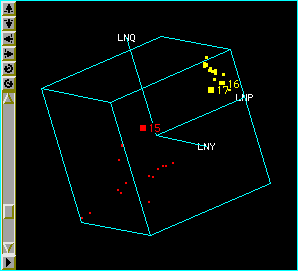# SAS/ETS Examples

## Chow Test for Structural Breaks

Contents | SAS Program

# OverviewPerhaps the most important assumption of any time series model is that the underlying process is the same across all observations in the sample. It is, therefore, necessary to analyze carefully time series data that include periods of violent change. A tool that is particularly useful in this regard is the Chow test.

The Chow test is commonly used to test for structural change in some or all of the parameters of a model in cases where the disturbance term is assumed to be the same in both periods.The Chow test is an application of the F-test, and it requires the sum of squared errors from three regressions - one for each sample period and one for the pooled data.

# Analysis

In an investigation of the demand for food in the United States, researchers may want to determine whether the structure of the demand equation changes after World War II.

## Exploring the Data Set

The data for this study include yearly observations on per capita food consumption, the price of food, and per capita income for the years 1927-1941 and 1948-1962 (Maddala 1992). There are no observations for the war years between 1942 and 1947. The DATA step creates a SAS data set named FOOD, reads data values into the variables YEAR, Q, P, and Y, and creates the constant term ONE and the log transformations LNQ, LNP, LNY.

   data food;
input year q p y @@;
retain one 1;
lnq = log(q);
lnp = log(p);
lny = log(y);
datalines;
27  88.9   91.7   57.7
28  88.9   92.0   59.3
29  89.1   93.1   62.0
...
;
run;


Once the FOOD data set is created, the interactive data analysis feature of SAS/INSIGHT software can be used to check the data for errors and to explore graphically possible relationships among the variables.

In this case, a 3-D Rotating Plot of the variables LNQ, LNP, and LNY show a break between the observations before 15 and after 16 corresponding to the years 1927-1941 and 1948-1962. This evidence suggests that a test for a structural break in a model for the demand for food may be appropriate.

## Computing the Chow Test

The AUTOREG procedure specifies a linear regression of the log of per capita food consumption on the log price of food, the log of per capita income, and a constant term (automatically included). The CHOW= option in the model statement performs Chow tests at the specified breakpoints. The breakpoint candidates 15, 16, and 17 corresponding to the years 1941, 1948, and 1949 are chosen from the preceding analysis.

   proc autoreg data=food;
model lnq = lnp lny / chow=(15 16 17);
run;


 CHOW Test

 The AUTOREG Procedure

 Dependent Variable lnq

 Ordinary Least Squares Estimates SSE 0.00286947 DFE 27 MSE 0.0001063 Root MSE 0.01031 SBC -182.30489 AIC -186.50848 Regress R-Square 0.9731 Total R-Square 0.9731 Durbin-Watson 1.2647

 Structural Change Test Test Break Point Num DF Den DF F Value Pr > F Chow 15 3 24 5.07 0.0074 Chow 16 3 24 5.54 0.0049 Chow 17 3 24 1.29 0.2992

 Variable DF Estimate Standard Error t Value Approx Pr > |t| Intercept 1 4.0473 0.1360 29.76 <.0001 lnp 1 -0.1189 0.0404 -2.95 0.0066 lny 1 0.2412 0.0134 17.95 <.0001

R2 is a measure for the appropriateness of the model. The value of 0.973 implies that LNP and LNY together explain more than 90% of the variation in LNQ.

The coefficient estimates are highly significant, while the negative sign on LNP and the positive sign on LNY satisfy the intuition that price elasticity has an inverse relationship to quantity demanded and income elasticity has a direct relationship.

Notice that the Chow test is highly significant for break points 15 and 16, which correspond to the years 1941 and 1948. This is not a very surprising result given the state of the world at that time.

# References

Chow, G.C. (1960), "Tests of Equality between Sets of Coefficients in Two Linear Regressions," Econometrica, 28, 591-605.

Fisher, F.M. (1970), "Tests of Equality between Sets of Coefficients in Two Linear Regressions: An Expository Note," Econometrica, 38, 361-366.

Greene, W.H. (1993), Econometric Analysis, Second Edition, New York: Macmillan Publishing Company.

Maddala, G.S. (1992), Introduction to Econometrics, Second Edition, New York: Macmillan Publishing Company.